# Implementing Attention Models in PyTorch

### Introduction:

Recurrent Neural Networks have been the recent state-of-the-art methods for various problems whose available data is sequential in nature. Adding attention to these networks allows the model to focus not only on the current hidden state but also take into account the previous hidden state based on the decoder’s previous output. There have been various different ways of implementing attention models. One such way is given in the PyTorch Tutorial that calculates attention to be given to each input based on the decoder’s hidden state and embedding of the previous word outputted. This article would introduce you to these mechanisms briefly and then demonstrate a different way of implementing attention that does not limit the number of input samples taken into consideration for calculating attention.

### Long Short Term Memory (LSTM):

Vanilla Recurrent Neural Networks fail to consider long term dependencies in various applications like language translators. Therefore, LSTMs were proposed to capture these long term dependencies. They have a memory cell which stores such long term dependencies and the hidden states are updated based on the update gates. The equations that govern the functioning of LSTM would make its working more clearer.

Some conventions used
x<ᵗ> input at time step ‘t’
a<ᵗ> hidden activation at time step ‘t’
c<ᵗ> memory cell at time step ‘t’
‘W’ trainable weights used for each operation
‘b’ trainable biases used for each operation.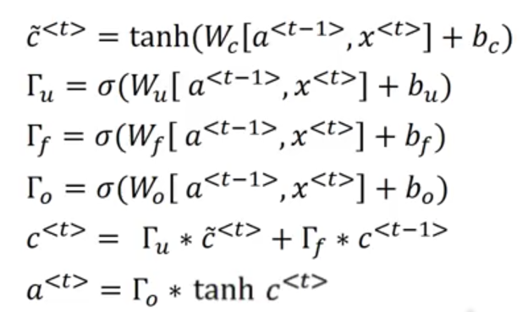Fig 1. LSTM equations

Here c̃<ᵗ> is the candidate value for updating the value of memory cell at time step ‘t’. This value is calculated based on activation from the previous time step and input from the current time step. Γꭉ, Γᵤ are two gates that determine if values from the previous memory cell are to be used or they are to be taken from the candidate values generated in the first equation. This helps the model to update values based on captured long-range dependencies. Note that, activation applied is sigmoid so that values of these stay very close to 0 or 1. If the value of the gate is 1 then that value is carried forward in the current memory cell else it’s not taken into consideration. In accordance with these gates, the current memory cell is updated by carrying out element-wise multiplication with respective vectors. Finally, the output is calculated as the element-wise multiplication of the Γₒ, that is the output gate, with the ‘tanh’ of the memory cell.

### Attention Networks:

Generally all sequence-to-sequence modeling tasks are modeled as two simple networks named as encoder and decoder where encoder takes the words as input and passes it through recurrent neural networks such as LSTMs or GRUs and the decoder uses this hidden state, and takes input a token such as <SOS> (Start Of Sentence) and output one token at a time, which is given as input for generation of next token word. Such models are used for various tasks like language translation, where, encoder takes in words in one language and decoder outputs the words of the desired language until <EOS> (End Of Sentence) token is outputted. See Fig  for the diagrammatic representation of models.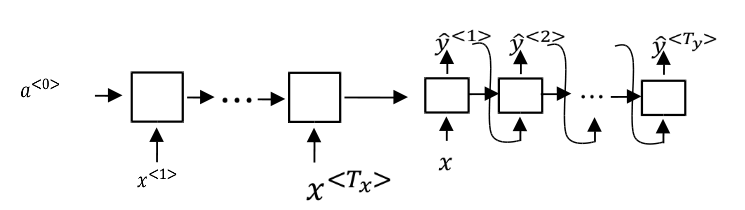Fig 2. Encoder Decoder model for Sequence to Sequence modeling

However, this model suffers when we try to use them for very long sequences. This happens, because we just consider the hidden state at the final step of the encoder for recovering the entire sequence of words at the decoder. Therefore, to avoid such problems, we use attention mechanisms which allow us to incorporate the hidden states at each input as the current hidden state by assigning them importance or ‘attention’. This method is quite intuitive as while translating into a particular language, a human translator tends to focus on certain words for predicting the next word rather than focusing on the entire sentence. Fig  shows the overview of attention mechanism. Note that, in Fig  we use a bidirectional LSTM. When we use bidirectional LSTMs we concatenate the output of each LSTM.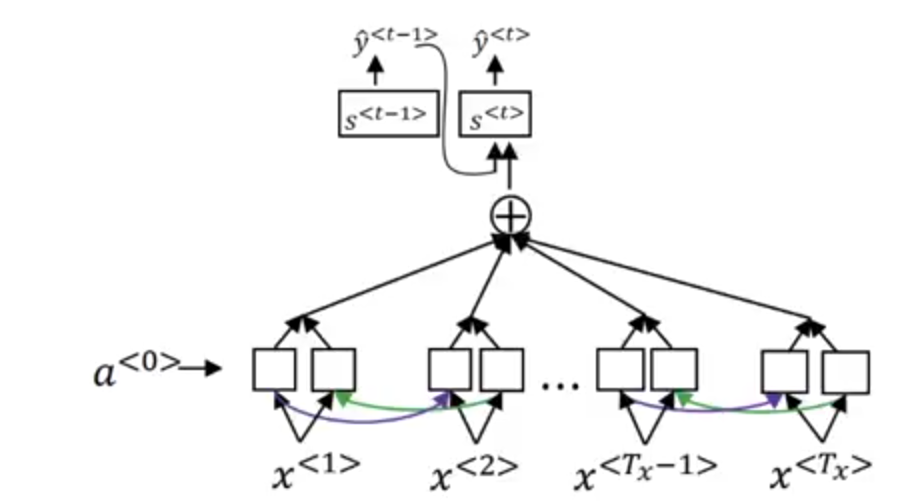Fig 3. Attention models: Intuition

The attention is calculated in the following way: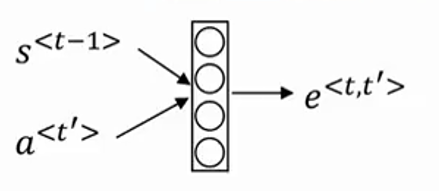Fig 4. Attention models: equation 1

an weight is calculated for each hidden state of each a<ᵗ’> with respect with decoder’s hidden state at time instant ‘t-1’ with the help of a small neural network.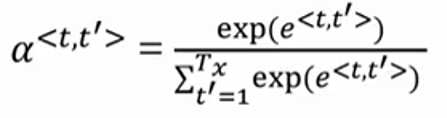Fig 5. Attention models: equation 2

Now, these weights then normalized using a softmax on values of e<ᵗ,ᵗ’> obtained from each of the input hidden state. These attention weights α<ᵗ,ᵗ’> signify the how much we need to ‘attend’ to this particular word at index t’ for predicting tᵗʰ word. We assign the input of the lstm as the concatenation of the weighted sum of all activations based on the attention weights (α<ᵗ,ᵗ’> * a<ᵗ>) and embedding of the previous word outputted.

### Diving into the code:

Some imports that we require to write the network.

This class is the Encoder for the attention network that is similar to the vanilla encoders. In the ‘__init__’ function we just store the parameters and create an LSTM layer. In the forward function, we just pass the input through the LSTM with the provided hidden state. The ‘init_hidden’ function is to be called before passing sentence through the LSTM to initialize the hidden state. Note that, the hidden state has to be two vectors, as LSTMs have two vectors i.e. hidden activation and the memory cell, in contrast with GRUs that is used in the PyTorch Tutorial. The first dimension of the hidden state is 2 for bidirectional LSTM (as bidirectional LSTMs are two LSTMs, one of which inputs the words in a forward manner, while the other one takes the words in reverse order) the second dimension is the batch size, which we take here to be 1 and the last one is the desired output size. Note that, I haven’t added any embedding for simplicity of the code.

This class is the attention based decoder that I have mentioned earlier. the ‘attn’ layer is used to calculate the value of e<ᵗ,ᵗ’> which is the small neural network mentioned above. This layer calculates the importance of that word, by using the previous decoder hidden state and the hidden state of the encoder at that particular time step. The ‘lstm’ layer takes in concatenation of vector obtained by having a weighted sum according to attention weights and the previous word outputted. The final layer is added to map the output feature space into the size of vocabulary, and also add some non-linearity while outputting the word. The ‘init_hidden’ function is used in the same way as in the encoder.

The forward function of the decoder takes the decoder’s previous hidden state, encoder outputs and the previous word outputted. ‘weights’ list is used to store the attention weights. Now, as we need to calculate attention weight for each encoder output, we iterate through them and pass them through the ‘attn’ layer along with decoder’s previous hidden state by concatenating them and store them in the ‘weights’ list. Once, we have these weights, we scale them in range (0,1) by applying softmax activation to them. To calculate the weighted sum, we use batch matrix multiplication to multiply attention vector of size (1,1, len(encoder_outputs)) and encoder_outputs of size (1, len(encoder_outputs), hidden_size) to obtain the size of vector hidden_size is the weighted sum. We pass the concatenation of obtained vector and the previous word outputted through the decoder LSTM, along with previous hidden states. The output of this LSTM is passed through the linear layer and mapped to vocabulary length to output actual words. We take argmax of this vector to obtain the word (the last step should be done in the main function).

For sake of testing the code, let’s create an encoder ‘c’ with an input size of 10 and output size of the encoder as 20 and make this LSTM bidirectional. We pass a random vector of size 10, and pass the hidden state to get output, as vectors ‘a’ and ‘b’. Note that, a.shape gives a tensor of size (1,1,40) as the LSTM is bidirectional; two hidden states are obtained which are concatenated by PyTorch to obtain eventual hidden state which explains the third dimension in the output which is 40 instead of 20. Also, the hidden state ‘b’ is a tuple two vectors i.e. the activation and the memory cell. The shape of each of these vectors is (2,1,20) (the first dimension is 2 due to the bidirectional nature of LSTMs).

Now we create an attention-based decoder with hidden size = 40 if the encoder is bidirectional, else 20 as we see that if they LSTM is bidirectional then outputs of LSTMs are concatenated, 25 as the LSTM output size and 30 as the vocab size. We pass a vector [a,a] i.e. two similar encoder outputs, just for the sake of understanding through the decoder. Also, assume that <SOS> token is all zeros. We see that shape of hidden state and output are (1,1,25) while weights are (0.5, 0.5) as we pass the same vector ‘a’ through the network.

Thus, using this model we could get text datasets, and use them for sequence to sequence modeling.

### Acknowledgement:

I would like to thank the Intel® Student Ambassador Program for AI, which provided me with the necessary training resources on the Intel® AI DevCloud and the technical support that helped me to use DevCloud.